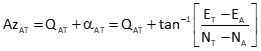## 3. AzimuthFigure F-2 Azimuth Observation

Equation F-4 is the angle of a line from the meridian in terms of a its coordinates.Equation F-4 A: At point T: To point αAT: angle from meridian QAT: quadrant addend

The angle from the meridian can be to the right (+) or to left (-) depending on the coordinate differences signs. This places the angle in one of the four quadrants, Figure F-3.Figure F-3 Angle from Meridian

The value of QAT, added to the angle to obtain the azimuth, depends on the quadrant. Table F-1 shows the sign of α, its quadrant and QAT value.

 Table F-1 Azimuth for a Line ij by QuadrantPartial derivatives of Equation F-4 are taken with respect to each coordinate of both points. The partial derivative for NF is:Remaining partial derivatives are:Equation F-5 is the azimuth observation equation, evaluated at initial coordinate approximations.Equation F-5Equation F-6